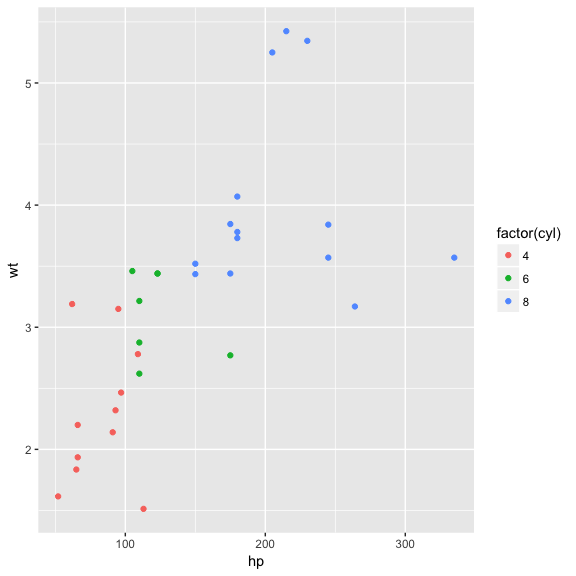# Introduction to ggconf

#### 2018-04-08

(This vignette is still in a draft).

ggplot2 provides a comprehensive collection of functions to draw a variety of informative plots.

ggplot(mtcars) +
geom_point(aes(x=hp, y=wt, colour=factor(cyl)))However, when finalizing plots for presentation (e.g. rotating labels, changing font sizes, or changing font families), ggplot2 needs long specifications of those appearance elements:

ggplot(mtcars) +
geom_point(aes(x=hp, y=wt, colour=factor(cyl)) +
labs(title = "Motor Cars Road Test") +
theme(plot.title = element_text(face = "bold"),
axis.text = element_text(face = "bold", size = 18),
axis.title.y = element_text(angle = 0),
plot.background = element_rect(fill="white"))

ggconf provides shorter and more flexible interface for modifying appearance elements:

ggplot(mtcars) +
geom_point(aes(x=hp, y=wt, colour=factor(cyl)) +
labs(title = "Motor Cars Road Test") +
theme(plot.title(face = "bold"), axis.text(face = "bold", size = 18),
axis.title.y(angle = 0), plot.bg(fill="white"))

Some people likes to write everything in a line. ggconf provides such a flexibility through ambiguous match capability:

ggplot(mtcars) +
geom_point(aes(x=hp, y=wt, colour=factor(cyl)) +
labs(title = "Motor Cars Road Test") +
theme(plt.ttl(f="bold"), a.txt(f="bold", z=18), a.ttl.y(angl=0), plt.bg(fl="white"))

## Why does the above command work?

One interesting thing about ggconf is the above functions are not actually functions:

# plot.title() is not defined

plot.title
Error: no such object 'plot.title'

The plot.title() function used above is undefined in R global environment. In fact, the above call are not a function, but just a sequence of symbols for compiling into a corresponding ggplot2 call.

## Debug Mode

If you define ggconf_debug, you could see verbose debugging message when calling theme2().